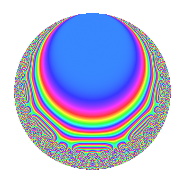# Properties

 Label 80.6.aLevel $80$ Weight $6$ Character orbit 80.a Rep. character $\chi_{80}(1,\cdot)$ Character field $\Q$ Dimension $10$ Newform subspaces $9$ Sturm bound $72$ Trace bound $3$

# Related objects

## Defining parameters

 Level: $$N$$ $$=$$ $$80 = 2^{4} \cdot 5$$ Weight: $$k$$ $$=$$ $$6$$ Character orbit: $$[\chi]$$ $$=$$ 80.a (trivial) Character field: $$\Q$$ Newform subspaces: $$9$$ Sturm bound: $$72$$ Trace bound: $$3$$ Distinguishing $$T_p$$: $$3$$

## Dimensions

The following table gives the dimensions of various subspaces of $$M_{6}(\Gamma_0(80))$$.

Total New Old
Modular forms 66 10 56
Cusp forms 54 10 44
Eisenstein series 12 0 12

The following table gives the dimensions of the cuspidal new subspaces with specified eigenvalues for the Atkin-Lehner operators and the Fricke involution.

$$2$$$$5$$FrickeDim.
$$+$$$$+$$$$+$$$$2$$
$$+$$$$-$$$$-$$$$3$$
$$-$$$$+$$$$-$$$$3$$
$$-$$$$-$$$$+$$$$2$$
Plus space$$+$$$$4$$
Minus space$$-$$$$6$$

## Trace form

 $$10q + 18q^{3} - 222q^{7} + 854q^{9} + O(q^{10})$$ $$10q + 18q^{3} - 222q^{7} + 854q^{9} + 604q^{11} - 450q^{15} + 1004q^{17} + 4416q^{19} - 820q^{21} - 4010q^{23} + 6250q^{25} + 9564q^{27} + 8052q^{29} - 756q^{31} - 1920q^{33} + 7350q^{35} - 10648q^{37} + 42948q^{39} - 10624q^{41} - 41918q^{43} + 5900q^{45} - 19582q^{47} + 45022q^{49} - 50540q^{51} - 42240q^{53} - 12100q^{55} - 60888q^{57} - 37000q^{59} + 36608q^{61} + 754q^{63} + 21100q^{65} + 70630q^{67} - 84764q^{69} - 64308q^{71} - 106196q^{73} + 11250q^{75} + 67376q^{77} - 22792q^{79} + 88118q^{81} + 229210q^{83} - 66200q^{85} - 34932q^{87} - 138108q^{89} + 24788q^{91} + 329192q^{93} - 72200q^{95} + 40772q^{97} - 32596q^{99} + O(q^{100})$$

## Decomposition of $$S_{6}^{\mathrm{new}}(\Gamma_0(80))$$ into newform subspaces

Label Dim. $$A$$ Field CM Traces A-L signs $q$-expansion
$$a_2$$ $$a_3$$ $$a_5$$ $$a_7$$ 2 5
80.6.a.a $$1$$ $$12.831$$ $$\Q$$ None $$0$$ $$-24$$ $$25$$ $$172$$ $$-$$ $$-$$ $$q-24q^{3}+5^{2}q^{5}+172q^{7}+333q^{9}+\cdots$$
80.6.a.b $$1$$ $$12.831$$ $$\Q$$ None $$0$$ $$-22$$ $$-25$$ $$-218$$ $$-$$ $$+$$ $$q-22q^{3}-5^{2}q^{5}-218q^{7}+241q^{9}+\cdots$$
80.6.a.c $$1$$ $$12.831$$ $$\Q$$ None $$0$$ $$-6$$ $$-25$$ $$118$$ $$-$$ $$+$$ $$q-6q^{3}-5^{2}q^{5}+118q^{7}-207q^{9}+\cdots$$
80.6.a.d $$1$$ $$12.831$$ $$\Q$$ None $$0$$ $$2$$ $$-25$$ $$62$$ $$+$$ $$+$$ $$q+2q^{3}-5^{2}q^{5}+62q^{7}-239q^{9}+\cdots$$
80.6.a.e $$1$$ $$12.831$$ $$\Q$$ None $$0$$ $$4$$ $$25$$ $$-192$$ $$-$$ $$-$$ $$q+4q^{3}+5^{2}q^{5}-192q^{7}-227q^{9}+\cdots$$
80.6.a.f $$1$$ $$12.831$$ $$\Q$$ None $$0$$ $$8$$ $$25$$ $$108$$ $$+$$ $$-$$ $$q+8q^{3}+5^{2}q^{5}+108q^{7}-179q^{9}+\cdots$$
80.6.a.g $$1$$ $$12.831$$ $$\Q$$ None $$0$$ $$18$$ $$-25$$ $$-242$$ $$+$$ $$+$$ $$q+18q^{3}-5^{2}q^{5}-242q^{7}+3^{4}q^{9}+\cdots$$
80.6.a.h $$1$$ $$12.831$$ $$\Q$$ None $$0$$ $$26$$ $$-25$$ $$22$$ $$-$$ $$+$$ $$q+26q^{3}-5^{2}q^{5}+22q^{7}+433q^{9}+\cdots$$
80.6.a.i $$2$$ $$12.831$$ $$\Q(\sqrt{129})$$ None $$0$$ $$12$$ $$50$$ $$-52$$ $$+$$ $$-$$ $$q+(6+\beta )q^{3}+5^{2}q^{5}+(-26+3\beta )q^{7}+\cdots$$

## Decomposition of $$S_{6}^{\mathrm{old}}(\Gamma_0(80))$$ into lower level spaces

$$S_{6}^{\mathrm{old}}(\Gamma_0(80)) \cong$$ $$S_{6}^{\mathrm{new}}(\Gamma_0(4))$$$$^{\oplus 6}$$$$\oplus$$$$S_{6}^{\mathrm{new}}(\Gamma_0(5))$$$$^{\oplus 5}$$$$\oplus$$$$S_{6}^{\mathrm{new}}(\Gamma_0(8))$$$$^{\oplus 4}$$$$\oplus$$$$S_{6}^{\mathrm{new}}(\Gamma_0(10))$$$$^{\oplus 4}$$$$\oplus$$$$S_{6}^{\mathrm{new}}(\Gamma_0(16))$$$$^{\oplus 2}$$$$\oplus$$$$S_{6}^{\mathrm{new}}(\Gamma_0(20))$$$$^{\oplus 3}$$$$\oplus$$$$S_{6}^{\mathrm{new}}(\Gamma_0(40))$$$$^{\oplus 2}$$